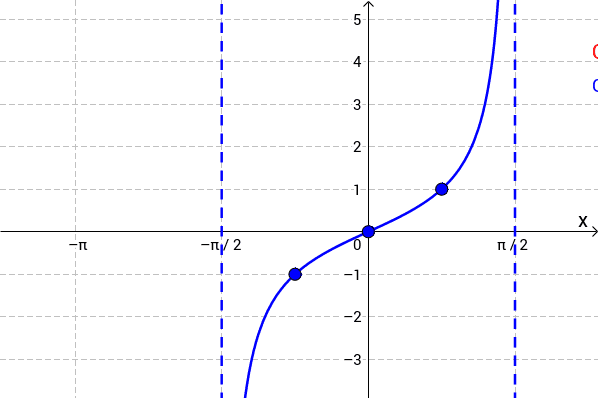# Tangent function

tan(x), tangent function.

## Tangent definition

In a right triangle ABC the tangent of α, tan(α) is defined as the ratio between the side opposite to angle α and the side adjacent to the angle α:

tan α = a / b

#### Example

a = 3"

b = 4"

tan α = a / b = 3 / 4 = 0.75

## Graph of tangent## Tangent rules

Rule name Rule
Symmetry

tan(-θ) = -tan θ

Symmetry tan(90°- θ) = cot θ
tan θ = sin θ / cos θ
tan θ = 1 / cot θ
Double angle tan 2θ = 2 tan θ / (1 - tan2 θ)
Angles sum tan(α+β) = (tan α + tan β) / (1 - tan α tan β)
Angles difference tan(α-β) = (tan α - tan β) / (1 + tan α tan β)
Derivative tan' x = 1 / cos2(x)
Integral ∫ tan x dx = - ln |cos x| + C
Euler's formula tan x = (eix - e-ix) / i (eix + e-ix)

## Inverse tangent function

The arctangent of x is defined as the inverse tangent function of x when x is real (x∈ℝ).

When the tangent of y is equal to x:

tan y = x

Then the arctangent of x is equal to the inverse tangent function of x, which is equal to y:

arctan x = tan-1 x = y

#### Example

arctan 1 = tan-1 1 = π/4 rad = 45°

See: Arctan function

## Tangent table

x

x

(°)

tan(x)
-π/2 -90° -∞
-1.2490 -71.565° -3
-1.1071 -63.435° -2
-π/3 -60° -√3
-π/4 -45° -1
-π/6 -30° -1/√3
-0.4636 -26.565° -0.5
0 0
0.4636 26.565° 0.5
π/6 30° 1/√3
π/4 45° 1
π/3 60° 3
1.1071 63.435° 2
1.2490 71.565° 3
π/2 90°

Currently, we have around 5611 calculators, conversion tables and usefull online tools and software features for students, teaching and teachers, designers and simply for everyone.